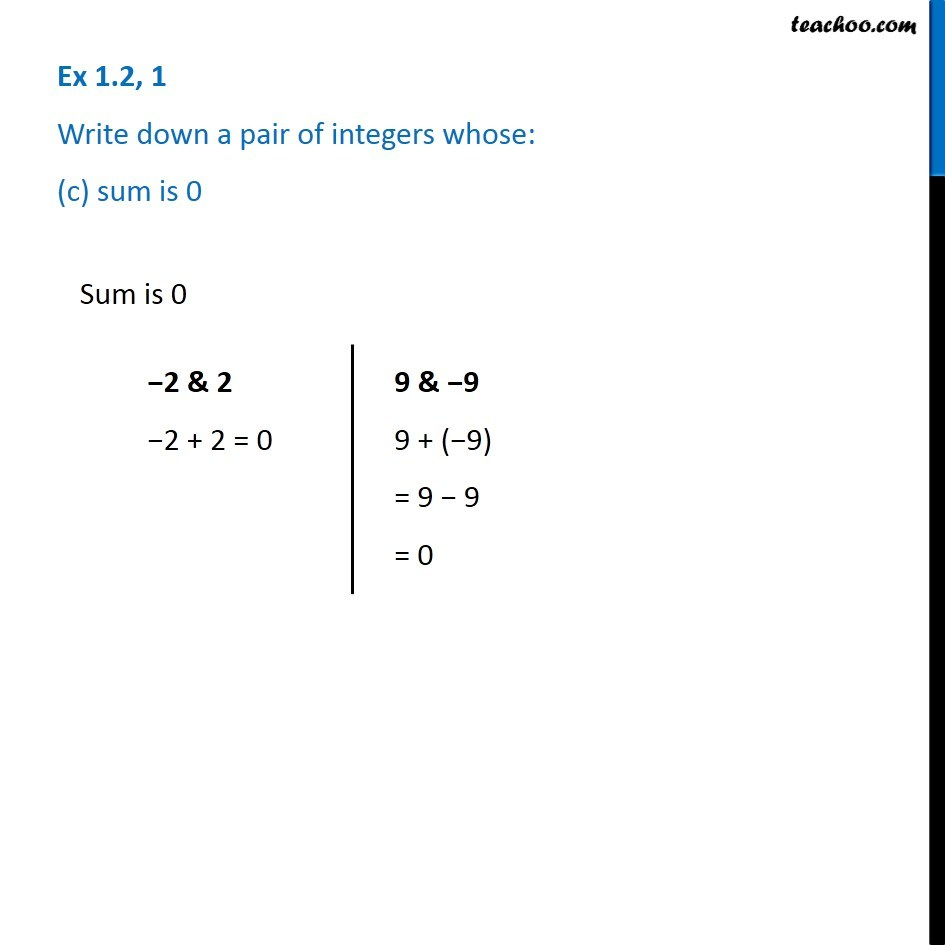1. Chapter 1 Class 7 Integers
2. Serial order wise
3. Ex 1.2

Transcript

Ex 1.2, 1 Write down a pair of integers whose: (c) sum is 0 Sum is 0 2 & 2 2 + 2 = 0 9 & 9 9 + ( 9) = 9 9 = 0